# Quantification of resolution

This section provides details of the solution obtained after running the diffuser in Alya. Firstly, details of the mesh resolution are given. Then, the obtained results are compared with those of the literature for the Stanford diffuser. A discussion on the closure of the Reynolds stress equations budgets follows and closes this section.

## Mesh resolution

The mesh resolution is quantified by obtaining a relation between the mesh characteristic length (${\Delta }$) and characteristic lengths of the turbulence, i.e., the Taylor microscale (${\eta _{T}}$) and Kolmogorov length scale (${\eta _{K}}$). The former relation is shown in Fig. 10 while the latter is reported in Fig. 11. As can be seen, both relations indicate that the resolution achieved by the present grid is at DNS level. In particular, it is commonly accepted that DNS is achieved when ${\Delta /\eta _{K}\leq 5}$, as shown in Fig. 11.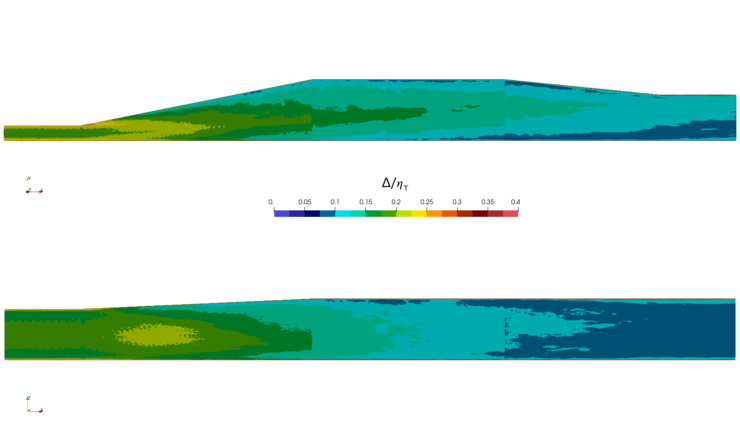Figure 10: Stanford double diffuser, Alya DNS-250M DoF, relation between the mesh size and the Taylor microscale.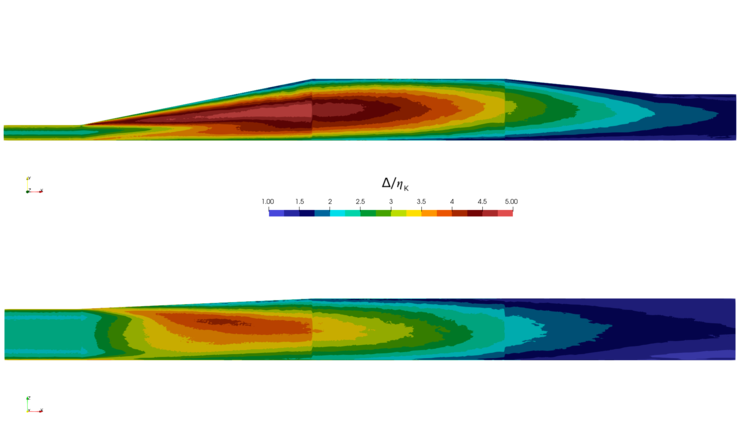Figure 11: Stanford double diffuser, Alya DNS-250M DoF, relation between the mesh size and the Kolmogorov length scale.

## Solution verification

For verification of the quality of the results obtained in the present simulations, these are compared with those of the DNS of Ohlsson et al. (2010) and the experimental data of Cherry et al. (2008) (available in UFR 4-16 of the Wiki) and a check is made whether the residuals in the budget equations for the Reynolds stresses are sufficiently small.

### Velocity profiles and RMS quantities

Figs. 16 and 17  presented in the next section (Statistical Data) show, respectively, the mean velocities and RMS values of the streamwise fluctuations along characteristic lines of the diffuser for the experimental data and both DNS simulations. Further,  Figs. 20 and 21 show, respectively, a comparison of the contours of the streamwise mean velocity and its RMS fluctuations at selected cross sections of the diffuser. As can be seen, there is a fair agreement between the 2 numerical simulations providing confidence in the present simulations, and where these deviate locally from the results of Ohlsson et al (2010),  the present results are closer to the experimental data of Cherry et al (2008)  so that overall good agreement is obtained with these data. An impression of the fluctuations can  be obtained from an animation that can be seen in this link. It should be noted that the inclusion of a roughness element in the long inlet duct in order to trigger turbulence has a negligible effect on the quality of the results.

### Reynolds stress equations budgets

The budget terms of the Reynolds-stress and turbulent kinetic energy (TKE) equations are discussed in this section. The first step is to show that Alya can close the budgets. To this aim, Figs. 12 shows the turbulent kinetic energy equation budget and the streamwise Reynolds-stress budget in a developed channel flow at ${Re_{\tau }=180}$. The results are compared with the DNS data of Hoyas and Jimenez (2008) (in circles) and Moser et al. (1999) (in triangles) obtained at the same ${Re_{\tau }}$. These are found freely available online. As can be seen, the residuals close well within an order of magnitude with respect to the production. This proves that the methodology used in this entry is sound and that Alya can close the budgets if given the necessary grid resolution and integration time.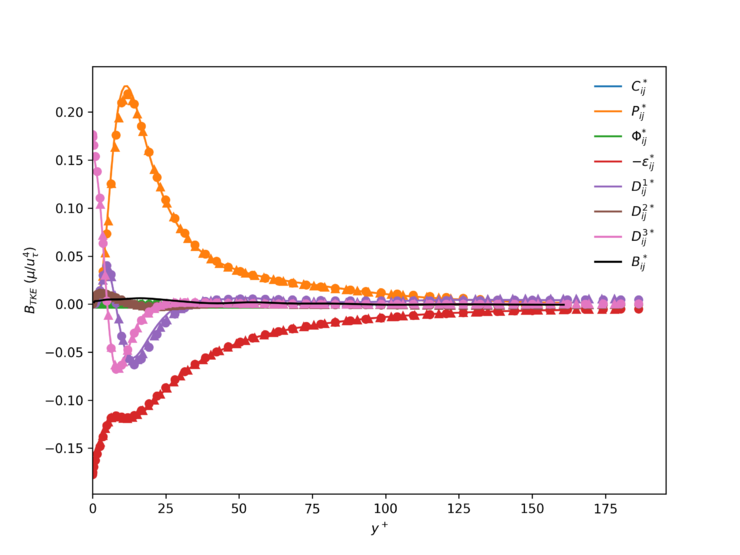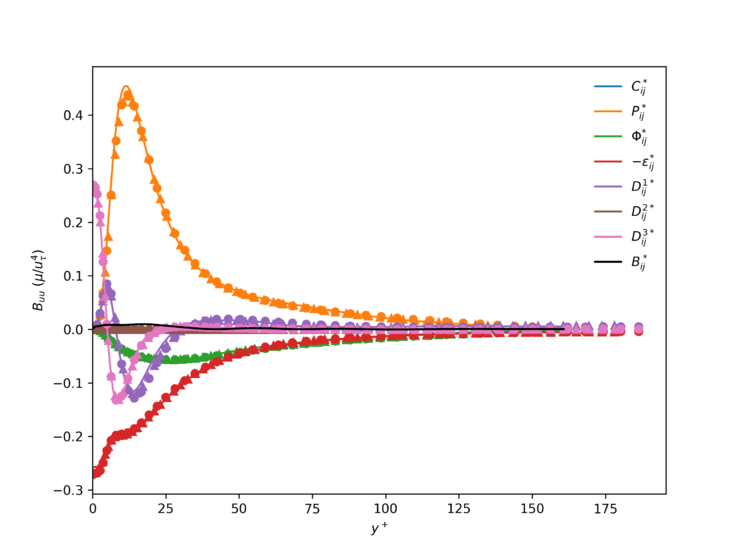Figure 12: Channel flow ${Re_{\tau }=180}$, Alya DNS-8M DoF, TKE and stream-wise Reynolds stress budget terms compared with the results of Hoyas and Jimenez (circles) and Moser, Kim and Mansour (triangles).

TKE and Reynolds-stress-budget profiles are provided in Statistical Data for the inlet duct along the vertical and horizontal mid lines. These profiles have been obtained by extracting the budget terms on representative sections between (${-30) for a total of a 100 planes every ${\Delta x/h=0.05}$. For each of these planes, pairs of slightly off-centered vertical (in y-direction) and horizontal ( in z-direction) lines (see Fig. 13) have been chosen along which the budget terms are extracted for a fixed x-position. This data has been averaged three times: 1) first averaging the data on each of the line pairs over the 100 x-positions; 2) then averaging the values at the 2 horizontal lines across the symmetry plane and likewise those of the two vertical lines; 3) folding the horizontal and vertical lines in one half of the duct at the middle lines onto the other half and then taking the average. The latter 2 averaging steps are also indicated in Fig. 13. This has been done to exploit the duct symmetries and reduce the amount of temporal instants required for convergence. These budget profiles are shown in Figs. 18 and 19. As can be seen, the budgets close reasonably well with residuals around 11.7% and 14.6% of the production for TKE for the vertical and horizontal lines respectively, and 10.4% and 12.2% of the production for the stream-wise Reynolds stress for the vertical and horizontal lines respectively.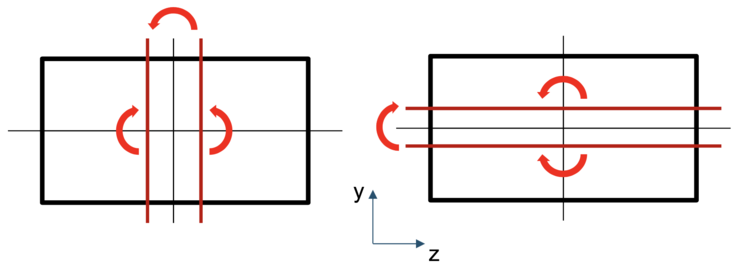Figure 13: Averaging steps for the duct region. Averaging the values at the 2 horizontal lines across the symmetry plane and likewise those of the two vertical lines, and folding the horizontal and vertical lines in one half of the duct at the middle lines onto the other half and then taking the average.

Regarding the budgets in the diffuser region, budget closure has been found to be more difficult to achieve. In that region massive separation occurs, and being in a full three-dimensional configuration, no symmetry can be exploited to diminish the time integration required to close the budgets. In addition to that, a "slow breathing" mechanism has been observed in the diffuser. This has been reported first by Malm et al. (2017) (note that due to a change of name Malm and Ohlsson are the same person) and was also observed in POD analysis conducted by the authors. In particular, a spanwise flapping motion has been observed inside the diffuser that corresponds to a period of 7 flow-through times. This means that this motion is only seen 3 times in both our and Ohlsson's 21 flow-through times and hence the budget data are not provided in the Statistical Data section for the diffuser area. As can be seen on Figs. 14 and 15, budget closure has not been achieved in the diffuser region. In order to start seeing some convergence, up to ${t=U_{b}/L=100}$(5 more times) integration time should be needed.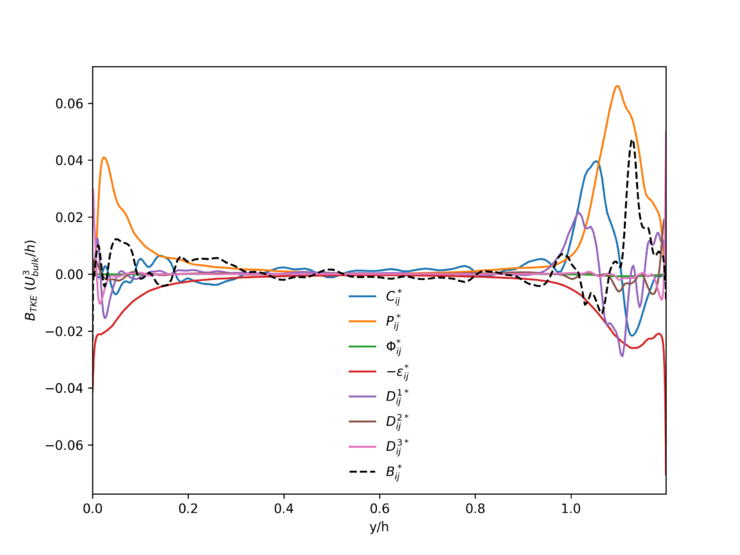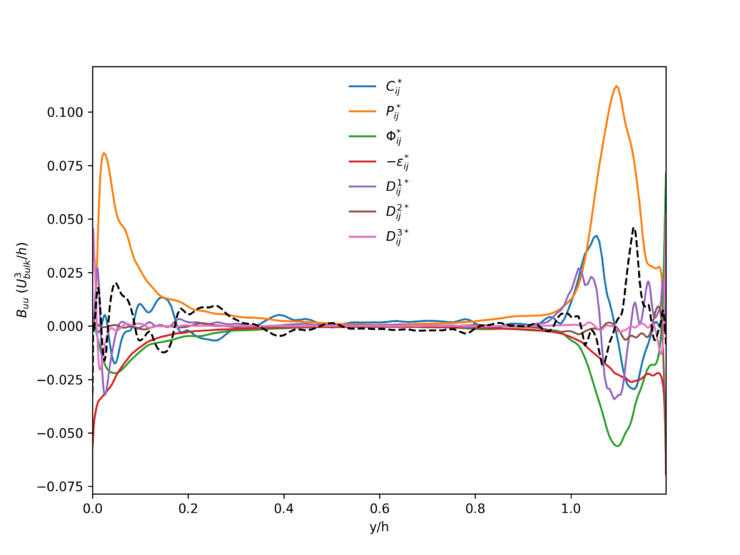Figure 14: Stanford double diffuser, Re=10000. TKE and stream-wise Reynolds stress budgets at the diffuser area (${x/h=1}$).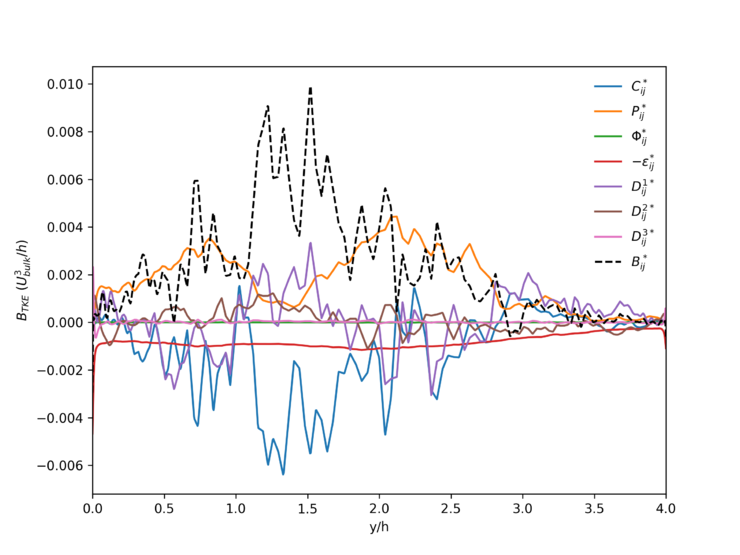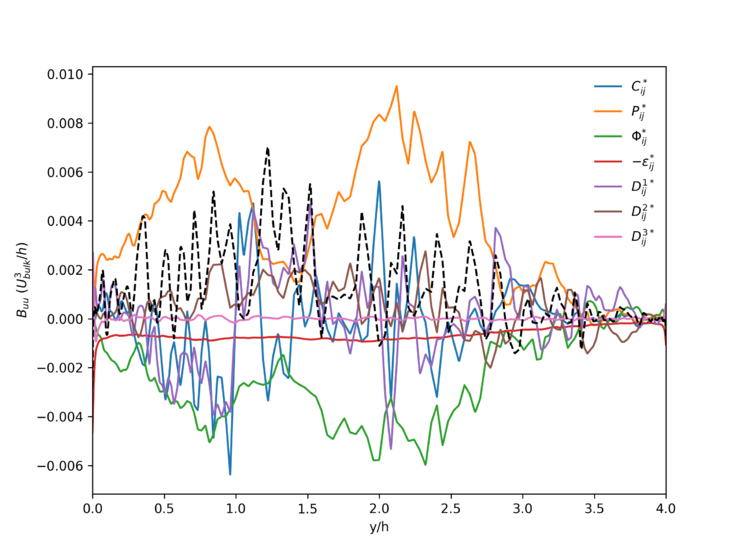Figure 15: Stanford double diffuser, Re=10000. TKE and stream-wise Reynolds stress budgets at the diffuser area (${x/h=15}$).

In the case of Figs. 14, smoothing does not help much since the physics are not well captured. Smoothing would likely distort the results and produce non-physical solutions. Therefore, it is recommended for future campaigns to increase further the grid resolution but also largely increase the integration time considered. While a DNS at Re=10000 is attainable, massively separated flows still pose a severe challenge regarding the computational effort due to the large integration time required to converge the solution in terms of the Reynolds stress budgets.